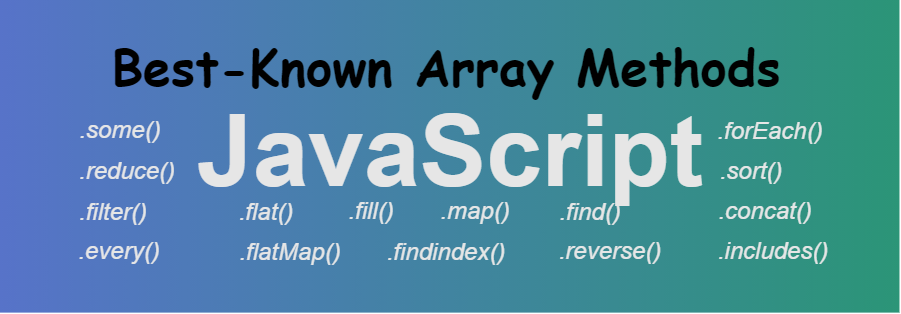Related Articles
Best-Known JavaScript Array Methods
• Difficulty Level : Basic
• Last Updated : 27 Jan, 2020An array is a special variable in all the languages that is used to store different elements. JavaScript array contains some built-in properties that every JavaScript developer should know how to use them and when or where to use them. We can use them to add, remove, iterate, or manipulate data as per our requirements. There are some basic JavaScript array methods that every developer should know.

1. some() Method: This method checks whether at least one of the elements of the array satisfies the condition checked by the argument function.

• Example:
 ``
• Output:
`true`

2. reduce() Method: The array reduce() method in JavaScript is used to reduce the array to a single value and executes a provided function for each value of the array (from left-to-right) and the return value of the function is stored in an accumulator.

• Example:
 ``
• Output:
`3`

3. map() Method: The map() method in JavaScript create an array by calling a specific function on each element present in the parent array. It is a non-mutating method. Generally, the map() method is used to iterate over an array and calling the function on every element of an array.

• Example:
 ``
• Output:
`2, 3, 4, 5`

4. every() Method: This method checks whether all the elements of the array satisfy the given condition or not that is provided by a function passed to it as the argument.

• Example:
 ` `
• Output:
`true`

5. flat() Method: This method creates a new array that contains more than arrays. Basically creates a simple array from an array that contains multiple arrays.

• Example:
 ` `
• Output:
`11, 89, 23, 7, 98`

6. flatMap() Method: This method is used to flatten the input array element into a new array. This method first of all map every element with the help of mapping function, then flattens the input array element into a new array.

• Example:
 `                    `
• Output:
`10, 20, 30, 40, 50`

7. filter() Method: This method is used to create a new array from a given array consisting of only those elements from the given array which satisfy a condition set by the argument function.

• Example:
 ``
• Output:
`112, 52, 944`

8. findindex() Method: This method returns index of the first element in a given array that satisfies the provided testing function. Otherwise -1 is returned.

• Example:
 ``
• Output:
`2`

9. find() Method: This method is used to get the value of the first element in the array that satisfies the provided condition. It checks all the elements of the array and whichever the first element satisfies the condition is going to print.

• Example:
 ` `
• Output:
`30`

10. fill() Method: This method used to fill the array with a given static value. The value can be used to fill the entire array or it can be used to fill a part of the array.

• Example:
 ` `
• Output:
`1, 87, 87, 58`

11. forEach() Method: This method calls the provided function once for each element of the array. The provided function may perform any kind of operation on the elements of the given array.

• Example:
 ` `
• Output:
`1, 841, 2209`

12. sort() Method: This method is used to sort the array. An array can be of any type i.e. string, numbers, characters etc.

• Example:
 `                    `
• Output:
`10, 25, 50, 88`

13. concat() Method: This method is used to merge two or more arrays together. This function does not alter the original arrays passed as arguments.

• Example:
 ` `
• Output:
`11, 12, 13, 14, 15, 16, 17, 18, 19`

14. includes() Method: This method is used to know either a particular element is present in the array or not and accordingly, it returns true or false i.e, if the element is present, then it returns true otherwise false

• Example:
 ` `
• Output:
`true`

15. reverse() Method: This method is used for in-place reversal of the array. The first element of the array becomes the last element and vice versa.

• Example:
 ` `
• Output:
```Original array: 34, 234, 567, 4
Newly reversed array: 4, 567, 234, 34```My Personal Notes arrow_drop_up